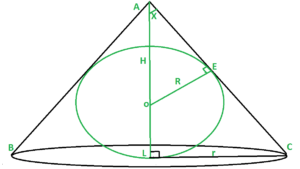# Minimum volume of cone that can be circumscribed about a sphere of radius R

Given a sphere of radius R, The task is to find out the minimum volume of the cone that can be circumscribed about it.Examples:

Input: R = 10
Output: Volume of cone = 8373.33
Explanation:
Radius of cone = 14.14 and Height of cone = 40,
Volume of cone =So, volume = 8373.33

Input: R = 4
Output: Volume of cone = 535.89


Approach:
we have given a sphere of radius R inscribed in Cone. We need to find out the radius and height of the cone to find out the volume of the cone.

1. In triangle AOE and ALC compute sin(X) i.e. For triangle AOEand for triangle ALC2. Now, From equating both we get3. Insert the value of H in Volume i.e.and for volume to be minimum.
4. From the above equation we getand putting this value in H we get5. Hence, applying the formula of volume of cone and puttingandwe get the desired result.

## C++

 // C++ program to find the minimum   // volume of the cone that can be    // circumscribed about a sphere  // of radius R  #include  using namespace std;     // Function to find the volume  // of the cone  float Volume_of_cone(float R)  {             // r = radius of cone      // h = height of cone      // Volume of cone = (1 / 3) * (3.14) * (r*r) * (h)      // we get radius of cone from the derivation      // is root(2) times multiple of R      // we get height of cone from the derivation      // is 4 times multiple of R      float V = (1 / 3.0) * (3.14) * (2 * ( R * R ) ) * (4 * R);             return V;  }         // Driver code   int main()  {      float R = 10.0;      cout << Volume_of_cone(R);  }          // This code is contributed by Samarth

## Java

 // Java program to find the minimum   // volume of the cone that can be   // circumscribed about a sphere  // of radius R  import java.util.*;     class GFG{     // Function to find the volume  // of the cone  static double Volume_of_cone(double R)  {             // r = radius of cone      // h = height of cone      // Volume of cone = (1 / 3) * (3.14) * (r*r) * (h)      // we get radius of cone from the derivation      // is root(2) times multiple of R      // we get height of cone from the derivation      // is 4 times multiple of R      double V = (double)((1 / 3.0) * (3.14) * (2 * (R * R)) *                                                     (4 * R));      return V;  }         // Driver code   public static void main(String[] args)  {      double R = 10.0;      System.out.print(Volume_of_cone(R));  }  }      // This code is contributed by sapnasingh4991

## Python3

 # Python3 program to find the minimum   # Volume of the cone that can be circumscribed   # about a sphere of radius R     import math      # Function to find the volume  # of the cone     def Volume_of_cone(R):          # r = radius of cone      # h = height of cone      # Volume of cone = (1 / 3) * (3.14) * (r**2) * (h)      # we get radius of cone from the derivation      # is root(2) times multiple of R      # we get height of cone from the derivation      # is 4 times multiple of R             V = (1 / 3) * (3.14) * (2 * ( R**2 ) ) * (4 * R)             return V            # Driver code   if __name__ == "__main__":              R = 10            print(Volume_of_cone(R))  

## C#

 // C# program to find the minimum   // volume of the cone that can be   // circumscribed about a sphere  // of radius R  using System;  class GFG{     // Function to find the volume  // of the cone  static double Volume_of_cone(double R)  {             // r = radius of cone      // h = height of cone      // Volume of cone = (1 / 3) * (3.14) * (r*r) * (h)      // we get radius of cone from the derivation      // is root(2) times multiple of R      // we get height of cone from the derivation      // is 4 times multiple of R      double V = (double)((1 / 3.0) * (3.14) *                      (2 * (R * R)) * (4 * R));      return V;  }         // Driver code   public static void Main()  {      double R = 10.0;      Console.Write(Volume_of_cone(R));  }  }      // This code is contributed by Nidhi_biet

Output:

8373.333333333332


Don’t stop now and take your learning to the next level. Learn all the important concepts of Data Structures and Algorithms with the help of the most trusted course: DSA Self Paced. Become industry ready at a student-friendly price.

My Personal Notes arrow_drop_upCheck out this Author's contributed articles.

If you like GeeksforGeeks and would like to contribute, you can also write an article using contribute.geeksforgeeks.org or mail your article to contribute@geeksforgeeks.org. See your article appearing on the GeeksforGeeks main page and help other Geeks.

Please Improve this article if you find anything incorrect by clicking on the "Improve Article" button below.

Practice Tags :

Be the First to upvote.

Please write to us at contribute@geeksforgeeks.org to report any issue with the above content.